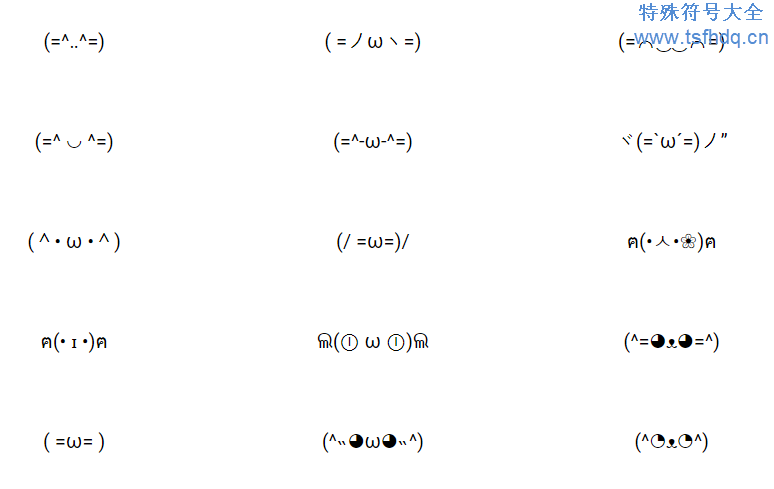# 可爱动物颜文字表情符号(=^･ω･^=)

(=^･ｪ･^=)

(=①ω①=)

( =ω=)..nyaa

(= ; ｪ ; =)

(=`ω´=)

(=^‥^=)

( =ノωヽ=)

(=⌒‿‿⌒=)

(=^ ◡ ^=)

(=^-ω-^=)

ヾ(=`ω´=)ノ”

(^• ω •^)

(/ =ω=)/

ฅ(•ㅅ•❀)ฅ

ฅ(• ɪ •)ฅ

ଲ(ⓛ ω ⓛ)ଲ

(^=◕ᴥ◕=^)

( =ω= )

(^˵◕ω◕˵^)

(^◔ᴥ◔^)

(^◕ᴥ◕^)

ต(=ω=)ต

( Φ ω Φ )

ฅ(^◕ᴥ◕^)ฅ